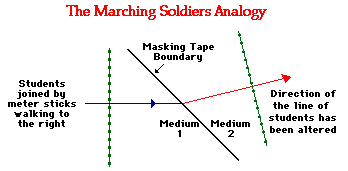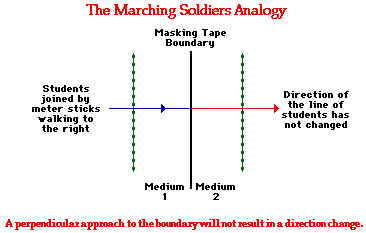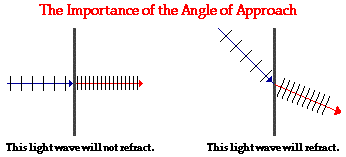Refraction and the Ray Model of Light - Lesson 1 - Refraction at a Boundary

# The Cause of Refraction

We have learned that refraction occurs as light passes across the boundary between two media. Refraction is merely one of several possible boundary behaviors by which a light wave could behave when it encounters a new medium or an obstacle in its path. The transmission of light across a boundary between two media is accompanied by a change in both the speed and wavelength of the wave. The light wave not only changes directions at the boundary, it also speeds up or slows down and transforms into a wave with a larger or a shorter wavelength. The only time that a wave can be transmitted across a boundary, change its speed, and still not refract is when the light wave approaches the boundary in a direction that is perpendicular to it. As long as the light wave changes speed and approaches the boundary at an angle, refraction is observed.

### The Marching Soldiers

But why does light refract? What is the cause of such behavior? And why is there this one exception to the refraction of light? An analogy of marching soldiers is often used to address this question. In fact, it is not uncommon that the analogy be illustrated in a Physics class with a student demonstration. A group of students forms a straight line (shoulder to shoulder) and connect themselves to their nearest neighbor using meter sticks. A strip of masking tape divides the room into two media. In one of the media (on one side of the tape), students walk at a normal pace. In the other media (or on the other side of the tape), students walk very slowly using baby steps. The group of students walk forward together in a straight line towards the diagonal strip of masking tape. The students maintain the line as they approach the masking tape. When an individual student reaches the tape, that student abruptly changes the pace of her/his walk. The group of students continues walking until all students in the line have entered into the second medium. The diagram below represents the line of students approaching the boundary (the masking tape) between the two media. On the diagram, an arrow is used to show the general direction of travel for the group of students in both media. Observe that the direction of the students changes at the "boundary."The fundamental feature of the students' motion that leads to this change in direction is the change in speed. Upon reaching the masking tape, each individual student abruptly changes speed. Because the students approach the masking tape at an angle, each individual student reaches the tape at a different time. The student who reaches the tape first, slows down while the rest of the line of students marches ahead. This occurs for every student in the line of students. Once a student reaches the boundary, that student slows down while his/her nearest neighbor marches ahead at the original pace. The result is that the direction that the line of students is heading is altered at the boundary. The change in speed of the line of students causes a change in direction.

### Conditions of Refraction

Will this refractive behavior always occur? No! There are two conditions that are required in order to observe the change in direction of the path of the students:

• The students must change speed when crossing the boundary.
• The students must approach the boundary at an angle; refraction will not occur when they approach the boundary head-on (i.e., heading perpendicular to it).

These are both reasonable enough conditions if you consider the previous paragraph. If the students do not change speed, then there is no cause factor. Recall that it was the change in speed of the students that caused the change in direction. The second condition is also reasonable. If the students approach the masking tape in a direction that is perpendicular to it, then each student will reach the tape at the exact same time. Recall that the line of student changed their direction because they had reached the masking tape at different times. The first student reached the tape, slowed down, and observed the rest of the students marching ahead at the original speed. The change in direction of the line of students only occurs at the boundary when the students change speed and approach at an angle.The Marching Soldiers analogy provides an excellent analogy to understanding the cause of light refraction. The line of students approaching the masking tape are analogous to a wavefront of light. The masking tape is analogous to a boundary between two media. The change in speed that occurred for the line of students would also occur for a wave of light. And like the marching students, a light wave will not undergo refraction if it approaches the boundary in a direction that is perpendicular to it.The same two conditions that are necessary for bending the path of the line of students are also necessary for bending the direction of a light ray. Light refracts at a boundary because of a change in speed. There is a distinct cause-effect relationship. The change in speed is the cause and the change in direction (refraction) is the effect.### Flickr Physics Photo

Laser light shown passing through a rectangular block of lucite. It enters the block along the normal line with an angle of incidence of 0°. There is no bending upon entering, nor upon leaving the block. When light travels along the normal to the boundary, it changes its speed but not its direction.### We Would Like to Suggest ...Why just read about it and when you could be interacting with it? Interact - that's exactly what you do when you use one of The Physics Classroom's Interactives. We would like to suggest that you combine the reading of this page with the use of our Refraction Interactive or our Least Time Principle Interactive. You can find these in the Physics Interactives section of our website. These Interactives provide the learner an interactive enivronment for exploring the refraction and/or reflection of light at a boundary between two materials.

Next Section:
Jump To Next Lesson:

Follow Us